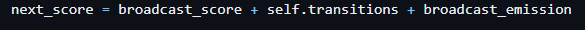# Viterbi algorithm implementation

Hello, I’m working on a RNN-CRF architecture for NLP task. I’ve used the CRF implementation provided by pytorch-crf — pytorch-crf 0.7.2 documentation, but I have a question about the implementation of Viterbi Algorthm.
Looking at the online implementations of the algorithm (for example Viterbi algorithm - Wikipedia) seems that the score (i,j) is computed using multiplication of emission score and transition score
while in the pytorch-crf they use sum to compute the next score:Can someone explain to me why? In pratice, do we have to do some assumptions?
Thanks a lot